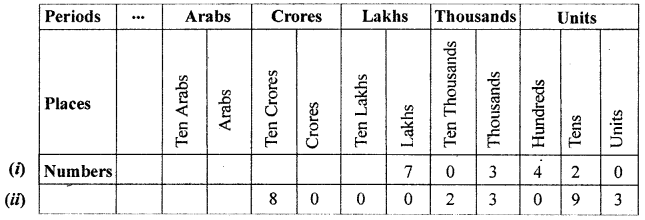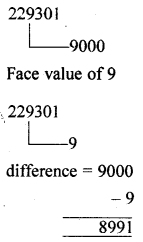## ML Aggarwal Class 6 Solutions for ICSE Maths Chapter 1 Knowing Our Numbers Ex 1.1

Question 1.
Write the smallest natural number. Can you write the largest natural number?
Solution:
Smallest natural number = 1
No, we can not write the largest natural number.

Question 2.
Fill in the blanks:
(i) 1 lakh = … ten thousand
(ii) 1 million = … hundred thousand
(iii) 1 crore = … ten lakh
(iv) 1 billion =… hundred million.
Solution:
(i) 1 lakh = ten ten thousand
(ii) 1 million = ten hundred thousand
(iii) 1 crore = ten ten lakh
(iv) 1 billion = ten hundred million.

Question 3.
Insert commas suitably and write each of the following numbers in words in the Indian system and the International system of numeration.
(i) 506723
(ii) 180018018
Solution:
(i) 506723 = 5,06,723
Five lakh six thousand seven hundred and twenty three.
(ii) 180018018 = 18,00,18,018
Eighteen crore eighteen thousand and eighteen.

Question 4.
Write the following numbers in expanded form:
(i) 750687
(ii) 5032109
Solution:
(i) 750687=700000 + 50000 + 600 + 80 + 7
(ii) 5032109 = 5000000 + 30000 + 2000 + 100 + 9

Question 5.
Write the following number in figures:
(i) Seven lakh three thousand four hundred twenty.
(ii) Eighty crore twenty three thousand ninety three.
Also write the above numbers in the place value chart.
Solution:
(i) 7,03,420
(ii) 80,00,23,093Question 6.
Write each of the following numbers in numeral form and place commas correctly:
(i) Seventy three lakh seventy thousand four hundred seven.
(ii) Nine crore five lakh forty one.
(iii) Fifty eight million four hundred twenty three thousand two hundred two.
Solution:
(i) 73,73,407
(ii) 9,05,00,041
(iii) 58,423,202

Question 7.
Write the face value and place value of the digit 6 in the number 756032.
Solution:
756032
Face value of 6 = 6
and place value = 6000

Question 8.
Find the difference between the place value and the face value of the digit 9 in the number 229301.
Solution:
Place value of 9Question 9.
Determine the difference of the place value of two 7’s in 37014472 and write it in words in International system.
Solution:
The given number in International system can be written as 37,014,472
The place value of 7 at ten’s place = 7 × 10 = 70
The place value of 7 at 7 million’s place = 7 × 1,000,000 = 7,000,000
The required difference = 7,000,000 – 70 = 6,999,930
Six million nine hundred ninty thousand nine hundred thirty.

Question 10.
Determine the product of place value and the face value of the digit 4 in the number 5437.
Solution:
Place value of 4Question 11.
Find the difference between the number 895 and that obtained on reversing its digits.
Solution:
First number = 895
Reversed number = 598
difference 297